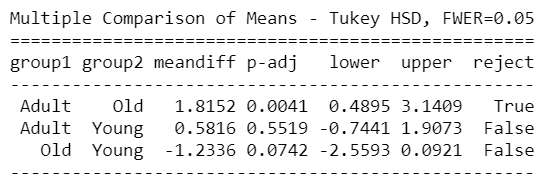# 方差分析介绍(结合COVID-19案例)

### 介绍

“事实是每个人都相信的简单陈述。也就是事实是没有错的，除非它被人发现了错误。假设有一个没人愿意相信的建议，那么它要直到被发现有效的时候才能成为事实。” –爱德华·泰勒### 什么是方差分析测试(ANOVA)

• 零假设–各组之间无显着差异
• 替代假设–各组之间存在显着差异

ANOVA公式的结果，即F统计量（也称为F比率），允许对多组数据进行分析，以确定样本之间和样本内部的可变性。### ANOVA检验的假设

1. 从因子水平定义的总体中独立且随机地获得观察结果
2. 每个因子水平的数据均呈正态分布
3. 案例独立性：样本案例应相互独立
4. 方差的同质性：同质性是指各组之间的方差应近似相等

### 方差分析检验类型

1. 单向方差分析：单向方差分析只有一个自变量
• 例如，可以按国家/地区评估日冕案例的差异，并且一个国家可以将2个，20个或更多不同的类别进行比较
2. 双向方差分析：双向方差分析（也称为因子方差分析）是指使用两个独立变量的方差分析
• 扩展上面的示例，双向方差分析可以按年龄组（独立变量1）和性别（独立变量2）检查日冕病例（因变量）的差异。双向方差分析可用于检查两个独立变量之间的相互作用。相互作用表明，自变量的所有类别之间的差异不是统一的
• 例如，老年组总体上可能比青年组具有更高的日冕病例，但是与欧洲国家相比，亚洲国家的差异可能更大（或更小）
3. N向方差分析：一个研究者也可以使用两个以上的自变量，这是一个N向方差分析（N是你拥有的自变量的数量），也就是MANOVA检验。
• 例如，可以同时按国家、性别、年龄组、种族等检查日冕病例的潜在差异
• 方差分析会给你一个单变量的f值，而方差分析会给你一个多变量的f值

#### 有复制与无复制

1. 具有复制功能的双向ANOVA：两个小组和这些小组的成员所做的不只是一件事情
• 例如，假设尚未开发出针对COVID-19的疫苗，医生正在尝试两种不同的治疗方法来治愈两组感染COVID-19的患者
2. 双向ANOVA（无复制）：只有一个组并且对同一组进行双重测试时使用
• 例如，假设已为COVID-19开发了一种疫苗，研究人员正在对一组志愿者进行疫苗接种之前和之后的测试，以查看其是否有效

### Python中的单向方差分析测试

import pandas as pd
import numpy as np

import scipy.stats as stats
import os
import random

import statsmodels.api as sm
import statsmodels.stats.multicomp

from statsmodels.formula.api import ols
from statsmodels.stats.anova import anova_lm
import matplotlib.pyplot as plt
from scipy import stats
import seaborn as sns


StatewiseTestingDetails=pd.read_csv('./StatewiseTestingDetails.csv')


StatewiseTestingDetails包含有关每个州一天中阳性和阴性病例总数的信息。而human_india_census2011包含有关每个州的密度的信息以及有关人口的其他相关信息。

population_india_census2011.head()


StatewiseTestingDetails['State'][StatewiseTestingDetails['Positive']==1].unique()


stateMedianData=StatewiseTestingDetails.groupby('State')[['Positive']].median().reset_index().rename(columns={'Positive':'Median'})

for index,row in StatewiseTestingDetails.iterrows():

if pd.isnull(row['Positive']):

StatewiseTestingDetails['Positive'][index]=int(stateMedianData['Median'][stateMedianData['State']==row['State']])

#合并StatewiseTestingDetails & population_india_census2011
data=pd.merge(StatewiseTestingDetails,population_india_census2011,on='State')


def densityCheck(data):
data['density_Group']=0
for index,row in data.iterrows():
status=None
i=row['Density'].split('/')
try:
if (',' in i):
i=int(i.split(',')+i.split(','))
elif ('.' in i):
i=round(float(i))
else:
i=int(i)
except ValueError as err:
pass
try:
if (0<i<=300):
status='Dense1'
elif (300<i<=600):
status='Dense2'
elif (600<i<=900):
status='Dense3'
else:
status='Dense4'
except ValueError as err:
pass
data['density_Group'].iloc[index]=status
return data


data=densityCheck(data)
#导出
stateDensity=data[['State','density_Group']].drop_duplicates().sort_values(by='State')df=pd.DataFrame({'Dense1':data[data['density_Group']=='Dense1']['Positive'],
'Dense2':data[data['density_Group']=='Dense2']['Positive'],
'Dense3':data[data['density_Group']=='Dense3']['Positive'],
'Dense4':data[data['density_Group']=='Dense4']['Positive']})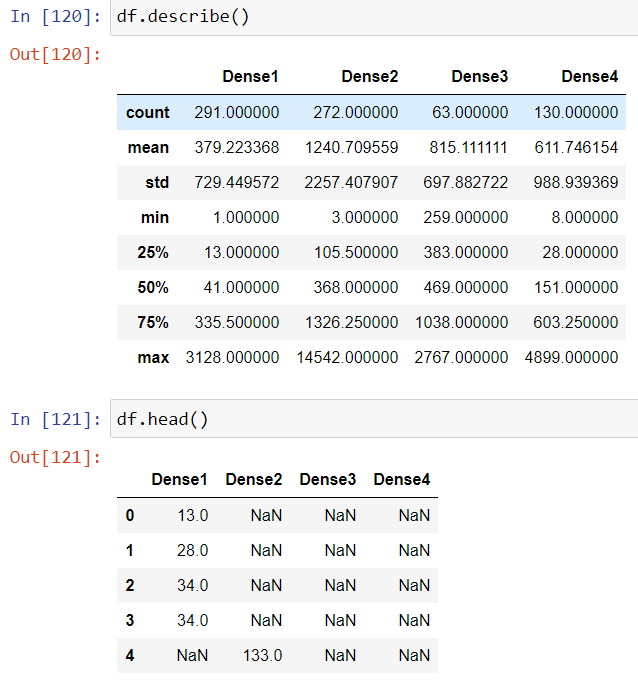np.random.seed(1234)
dataNew=pd.DataFrame({'Dense1':random.sample(list(data['Positive'][data['density_Group']=='Dense1']), 10),
'Dense2':random.sample(list(data['Positive'][data['density_Group']=='Dense1']), 10),
'Dense3':random.sample(list(data['Positive'][data['density_Group']=='Dense1']), 10),
'Dense4':random.sample(list(data['Positive'][data['density_Group']=='Dense1']), 10)})dataNew['Dense1'],fitted_lambda = stats.boxcox(dataNew['Dense1'])
dataNew['Dense2'],fitted_lambda = stats.boxcox(dataNew['Dense2'])
dataNew['Dense3'],fitted_lambda = stats.boxcox(dataNew['Dense3'])
dataNew['Dense4'],fitted_lambda = stats.boxcox(dataNew['Dense4'])#### 方法1：使用statsmodels模块进行单向方差分析

Python中有两种方法可以执行ANOVA测试。一个是stats.f_oneway()方法：

F, p = stats.f_oneway(dataNew['Dense1'],dataNew['Dense2'],dataNew['Dense3'],dataNew['Dense4'])
## 看看整体模型是否重要
print('F-Statistic=%.3f, p=%.3f' % (F, p))


#### 方法2：用OLS模型进行单因素方差分析

model = ols('Count ~ C(density_Group)', newDf).fit()
model.summary()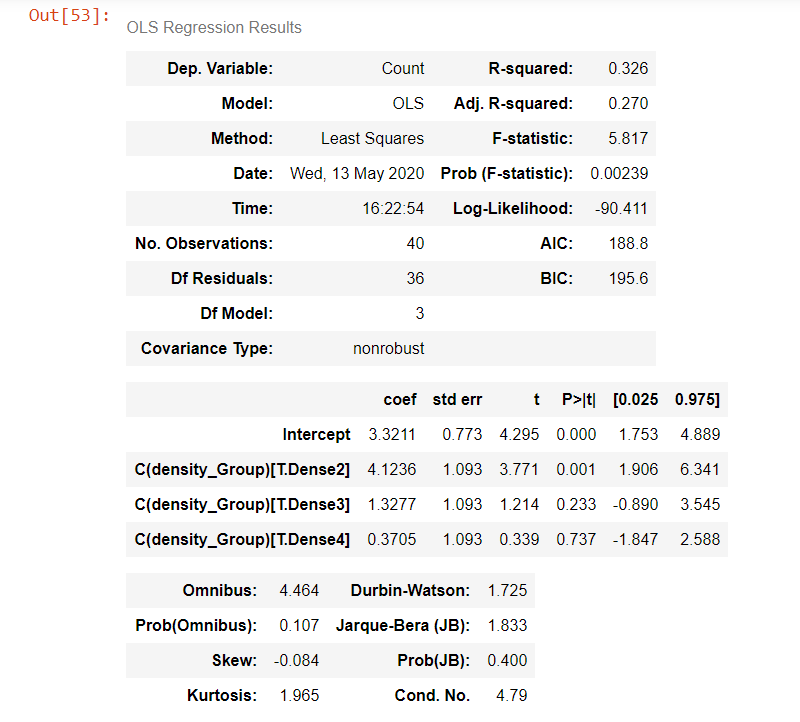## 看看整体模型是否重要
print(f"Overall model F({model.df_model: .0f},{model.df_resid: .0f}) = {model.fvalue: .3f}, p = {model.f_pvalue: .4f}")

#创建方差分析表
res = sm.stats.anova_lm(model, typ= 2)
res


F-statistic= 5.817，p-value= 0.002，这表明density_Group对日冕阳性病例有总体显着影响。但是，我们尚不知道desnity_groups之间的区别在哪里。因此，基于p值，我们可以拒绝H0；就面积密度和日冕例数而言，没有显着差异。

#### 事后比较检验

mc = statsmodels.stats.multicomp.MultiComparison(newDf['Count'],newDf['density_Group'])
mc_results = mc.tukeyhsd()
print(mc_results)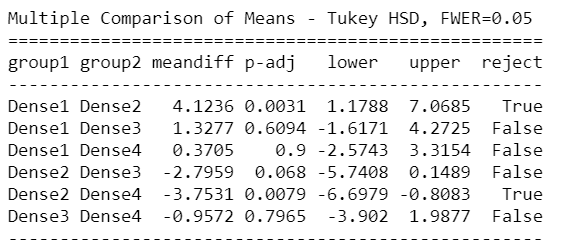Tuckey HSD测试清楚地表明，Group1 – Group3，Group1 – Group4，Group2 – Group3和Group3 – Group4之间存在显着差异。

#### 正态分布假设检验

##### 方法1:Shapiro-Wilk试验：
### 正态性假设检查
w, pvalue = stats.shapiro(model.resid)
print(w, pvalue)


##### 方法2:Q-Q图试验：

res = model.resid
fig = sm.qqplot(res, line='s')
plt.show()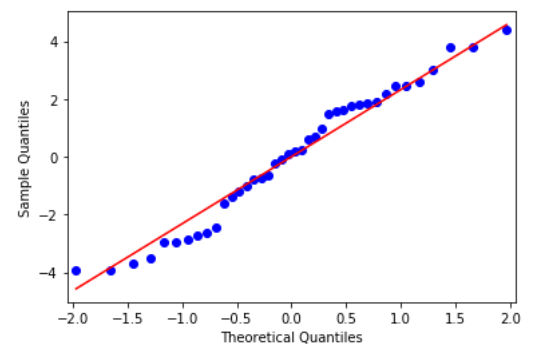#### 方差假设检验的同质性检查

w, pvalue = stats.bartlett(newDf['Count'][newDf['density_Group']=='Dense1'], newDf['Count'][newDf['density_Group']=='Dense2']
, newDf['Count'][newDf['density_Group']=='Dense3'], newDf['Count'][newDf['density_Group']=='Dense4'])
print(w, pvalue)

## Levene 方差测试
stats.levene(dataNew['Dense1'],dataNew['Dense2'],dataNew['Dense3'],dataNew['Dense4'])


### Python中的双向方差分析测试

individualDetails=pd.read_csv('./individualDetails.csv',parse_dates=)individualDetails.isna().sum()

print('Percentage of missing values in age & gender columns respectively :',
(individualDetails['age'].isna().sum()/individualDetails.shape)*100,'%',
(individualDetails['gender'].isna().sum()/individualDetails.shape)*100,'%')


ageMedianPerState=individualDetails[~individualDetails['age'].isna()]
ageMedianPerState['age']=ageMedianPerState['age'].astype(str).astype(int)
ageMedianPerState=ageMedianPerState.groupby('State')[['age']].median().reset_index()
ageMedianPerState['age']=ageMedianPerState['age'].apply(lambda x:math.ceil(x))

#通过COVID-19查找每个州的最常感染的性别
genderModePerState=individualDetails.groupby(['State'])['gender'].agg(pd.Series.mode).to_frame().reset_index()

#这没有获得有关总体性别的信息性别

#现在在年龄和性别栏填充丢失的值
for index,row in individualDetails.iterrows():
individualDetails.drop([index],inplace=True)
continue
if pd.isnull(row['age']):
individualDetails['age'][index]=list(ageMedianPerState['age'][ageMedianPerState['State']=='West Bengal'].values)
if pd.isnull(row['gender']):
if len(genderModePerState['gender'][genderModePerState['State']==row['State']].values)>0:
individualDetails['gender'][index]=(genderModePerState['gender'][genderModePerState['State']==row['State']].values)



data = pd.merge(individualDetails,stateDensity,on='State',how='left').reset_index(drop=True)


data.dropna(subset=['density_Group'],inplace=True)
data.reset_index(drop=True,inplace=True)


patient_Count=data.groupby(['diagnosed_date','density_Group'])[['diagnosed_date']].count().

rename(columns={'diagnosed_date':'Count'}).reset_index()

data=pd.merge(data,patient_Count,on=['diagnosed_date','density_Group'],how='inner')

newData=data.groupby(['density_Group','age_Group'])['Count'].apply(list).reset_index()

np.random.seed(1234)

for index,row in newData.iterrows():
count=17
tempDf=pd.DataFrame(index=range(0,count),columns=['density_Group','age_Group','Count'])

tempDf['age_Group']=newData['age_Group'][index]

tempDf['density_Group']=newData['density_Group'][index]

tempDf['Count']=random.sample(list(newData['Count'][index]),count)



plt.hist(AnovaData['Count'])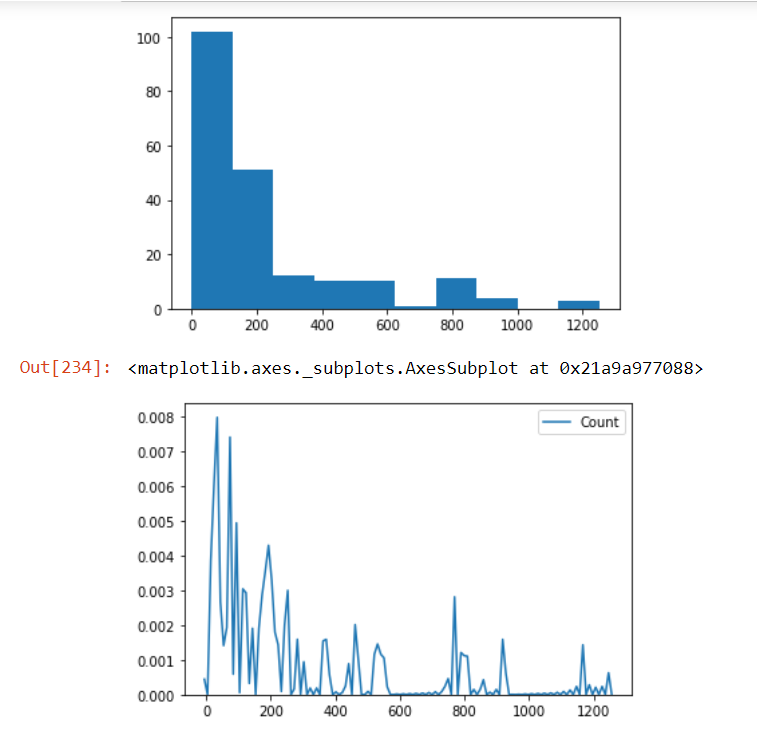sns.boxplot(x='age_Group', y='Count',
palette="colorblind")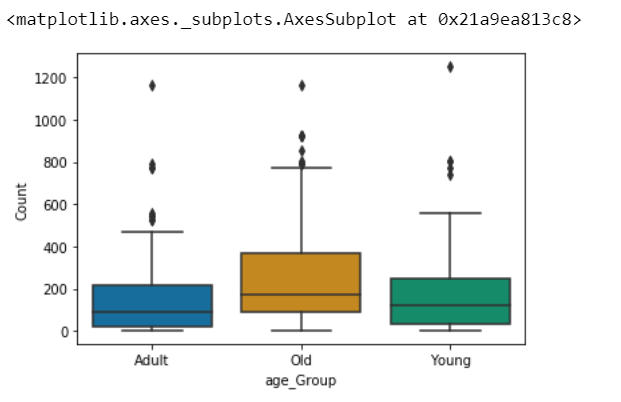AnovaData['Count']=AnovaData['Count'].astype(int)

## 数据转换

import matplotlib.pyplot as plt


## 拟合OlS模型-方法1
model2 = ols('newCount ~ C(age_Group)+ C(density_Group)', AnovaData).fit()

print(f"Overall model F({model2.df_model: },{model2.df_resid: }) = {model2.fvalue: }, p = {model2.f_pvalue: }")
model2.summary()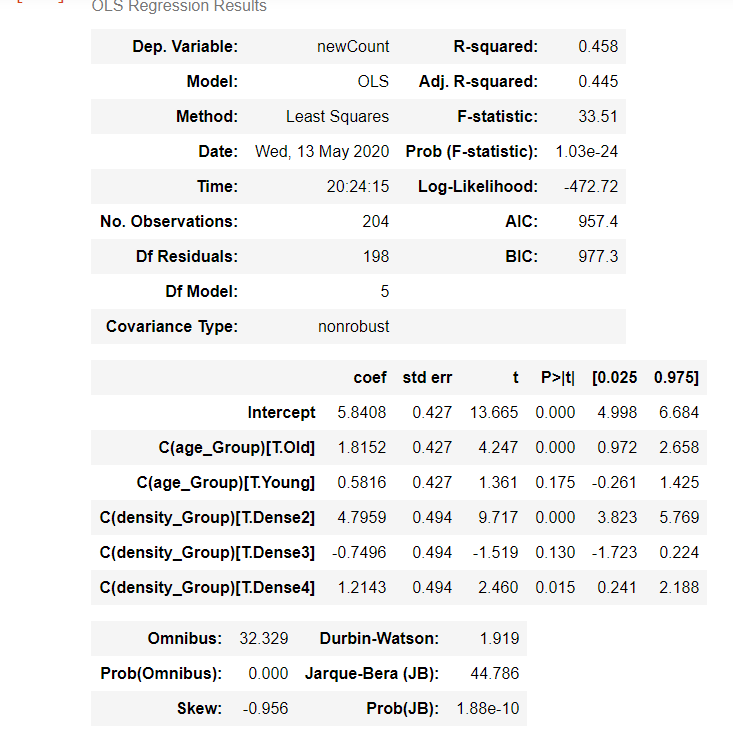## 创建方差分析表
res2 = sm.stats.anova_lm(model2, typ=2)
res2

#检验残差的正态分布
res = model2.resid
fig = sm.qqplot(res, line='s')
plt.show()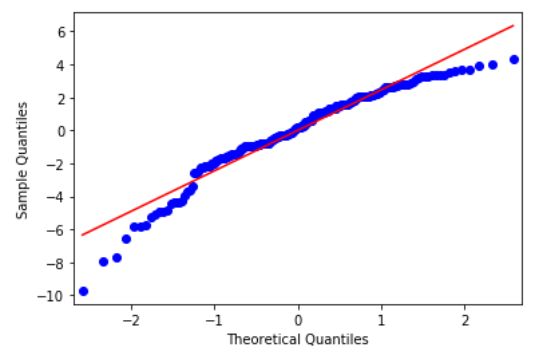#方法2-检查组之间的交互
formula = 'newCount ~ C(age_Group) *C(density_Group)'
model.summary()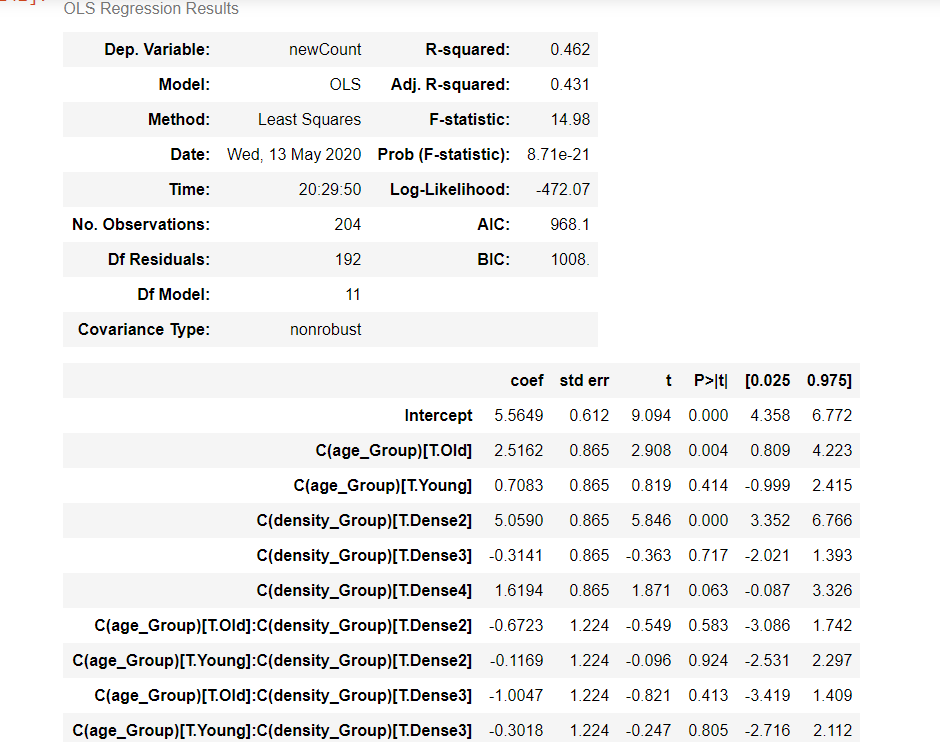aov_table = anova_lm(model, typ=2)
print(aov_table.round(4))


#### 结果的解释

age_Group显着影响日冕病例的结果，age_Group和density_Group的相互作用也显着影响日冕病例的结果。

#### 事后检验

mc = statsmodels.stats.multicomp.MultiComparison(AnovaData['newCount'],AnovaData['density_Group'])
mc_results = mc.tukeyhsd()
print(mc_results)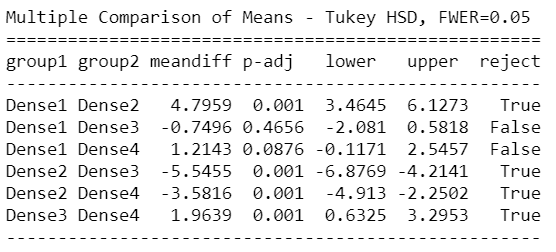mc = statsmodels.stats.multicomp.MultiComparison(AnovaData['newCount'],AnovaData['age_Group'])
mc_results = mc.tukeyhsd()
print(mc_results)